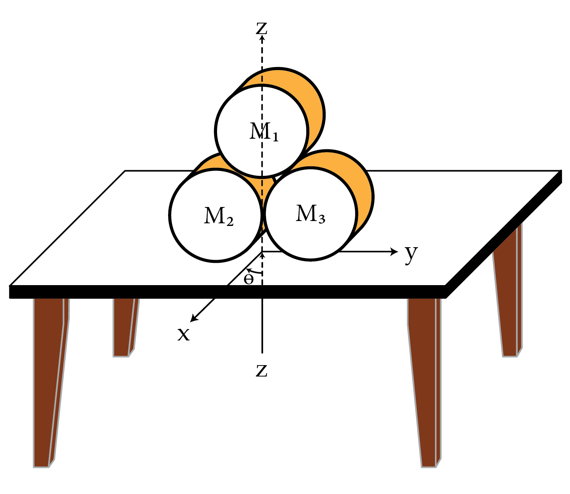Stacking cylindersThree cylinders, all of the same mass, are stacked on a table as shown in the figure. There is enough friction between the cylinders and the table such that the cylinders remain at rest.

Let $F_h$ and $F_n$, respectively, be the horizontal and the normal forces of the table on one of the cylinders. When $F_h$ is a minimum, what is the ratio $F_h/F_n$?

Note:

The z-axis is vertical, x and y-axis are along the table. The angle $\theta$ shown in the diagram is $90^\circ$.

×